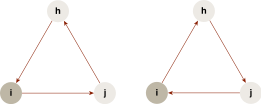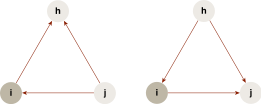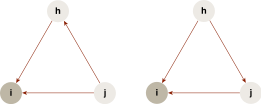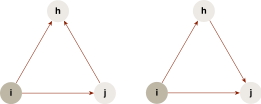# 1.1 - 8.10 - Unweighted, Directed Network (BDN) - Teradata Vantage

## Teradata Vantage™ - Machine Learning Engine Analytic Function Reference

Product
Release Number
1.1
8.10
Published
October 2019
Content Type
Programming Reference
Publication ID
B700-4003-079K
Language
English (United States)

For an unweighted, directed network (BDN), given a vertex i, the BDN triangle types can be categorized to four patterns.

Triangle Type Patterns
Pattern Description Illustration
Cycle There is a cyclical relation among i and any two of its neighbors: ijhi, or the reverse.Middleman One of i’s neighbors, j, both holds an outward edge to a third neighbor, h, and uses i to reach h in two steps.In i holds two inward edges.Out i holds two outward edges.For each pattern, this is the formula for the clustering coefficient (CC):

c i * = δ i * / τ i *

where {*}={cycle, middleman, in, out}.

These are the triples for each pattern:

τ i cyc = d i in d i out - d i

where d i is the number of bilateral edges between i and its neighbors.

τ i mid = d i in d i out - d i

τ i in = d i in (d i in - 1)

τ i out = d i out (d i out - 1)